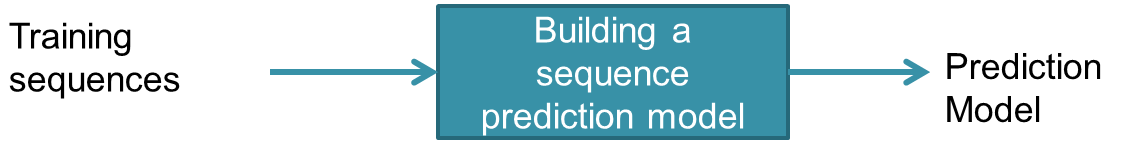# An Introduction to Sequence Prediction

In this blog post, I will give an introduction to the task of sequence prediction,  a popular data mining/machine learning task, which consist of predicting the next symbol of a sequence of symbols. This task is important as it have many real-life applications such as webpage prefetching and product recommendation.

What is a sequence?

Before defining the problem of sequence prediction, it is necessary to first explain what is a sequence. A sequence is an ordered list of symbols. For example, here are some common types of sequences:

• A sequence of webpages visited by a user, ordered by the time of access.
• A sequence of words or characters typed on a cellphone by a user, or in a text such as a book.
• A sequence of products bought by a customer in a retail store
• A sequence of proteins in bioinformatics
• A sequence of symptoms observed on a patient at a hospital

Note that in the above definition, we consider that a sequence is a list of symbols and do not contain numeric values.  A sequence of numeric values is usually called a time-series rather than a sequence, and the task of predicting a time-series is called time-series forecasting. But this is another topic.

What is sequence prediction?

The task of sequence prediction consists of predicting the next symbol of a sequence based on the previously observed symbols. For example, if a user has visited some webpages A, B, C, in that order, one may want to predict what is the next webpage that will be visited by that user to prefetch the webpage.An illustration of the problem of sequence prediction

There are two steps to perform sequence prediction:

1. First,  one must train a sequence prediction model using some previously seen sequences called the training sequences.  This process is illustrated below:For example, one could train a sequence prediction model for webpage prediction using the sequences of webpages visited by several users.

2. The second step is to use a trained sequence prediction model to perform prediction for new sequences (i.e. predict the next symbol of a new sequence), as illustrated below.For example, using a prediction model trained with the sequences of webpages visited by several users, one may predict the next webpage visited by a new user.

An overview of state-of-the-art sequence prediction models

Having defined what are the main sequence prediction models, that could be used in an application?

There are actually numerous models that have been proposed by researchers such as DG, All-k-order Markov, TDAG, PPM, CPT and CPT+.  These models utilize various approaches. Some of them uses for example neural networks, pattern mining and a probabilistic approach.

How to determine if a sequence prediction model is good?

Various sequence prediction models have different advantages and limitations, and may perform more or less well on different types of data. Typically a sequence prediction model is evaluated in terms of criteria such as prediction accuracy, the memory that it uses and the execution time for training and performing predictions.

Several benchmark have been done in the literature to compare various models. For example, here is a recent benchmark performed by my team in our PAKDD 2015 paper about sequence prediction with CPT+In this benchmark, we compare our proposed CPT+ sequence prediction model with several state-of-the-art models on various types of data.  Briefly, BMS, MSNBC, Kosarak and Fifa are sequences of webpages. SIGN is a sign-language dataset. Bible word and Bible char are datasets of sequences of Words and characters. As it can be seen, for this type of data at least, CPT+ greatly outperform other models.  There are several reasons. One of them is that several models such as DG assume the Markovian hypothesis that the next symbol only depends on the previous symbols. Another reason is that the CPT+ model use an efficient indexing approach to consider all the relevant data for each prediction (see details in the paper).

Where can I get open-source implementations of sequence prediction models?

Some open-source and Java implementation of the seven discussed sequence prediction models (DG, AKOM, TDAG, LZ78, PPM, CPT, CPT+) can be found in the SPMF open-source data mining library, which includes the implementations from the IPredict project.

There are extensive documentation about how to use these models on the SPMF website. Here I will provide a quick example that shows how the CPT model can be easily applied with just a few lines of code.

```               // Phase 1:  Training
// Load a file containing the training sequences into memory
SequenceDatabase trainingSet = new SequenceDatabase();

// Train the prediction model
String optionalParameters = "splitLength:6 recursiveDividerMin:1 recursiveDividerMax:5";
CPTPredictor predictionModel = new CPTPredictor("CPT", optionalParameters);
predictionModel.Train(trainingSet.getSequences());

// Phase 2: Sequence prediction
// We will predict the next symbol after the sequence <1,4>
Sequence sequence = new Sequence(0);